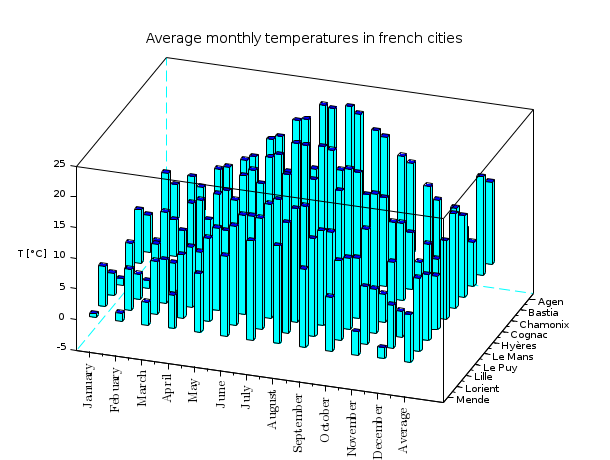Scilab Home page | Wiki | Bug tracker | Forge | Mailing list archives | ATOMS | File exchange
Change language to: Français - Português - 日本語 - Русский

See the recommended documentation of this function

Scilab Help >> Graphics > 3d_plot > hist3d

# hist3d

3D representation of a histogram

### Syntax

```hist3d(mtx,[theta,alpha,leg,flag,ebox])
hist3d(mtx,<opt_args>)
hist3d(list(mtx,x,y),[theta,alpha,leg,flag,ebox])
hist3d(list(mtx,x,y),<opt_args>)```

### Arguments

mtx

matrix of size (m,n) defining the histogram `mtx(i,j)=F(x(i),y(j))`, where `x` and `y` are taken as `0:m` and `0:n`.

list(mtx,x,y)

where mtx is a matrix of size (m,n)defining the histogram `mtx(i,j)=F(x(i),y(j))`, with `x` and `y` vectors of size (1,m+1) and (1,n+1).

<opt_args>

This represents a sequence of statements ```key1=value1, key2=value2``` ,... where `key1`, `key2,...` can be one of the following: theta, alpha,leg,flag,ebox. See plot3d.

theta,alpha,leg,flag,ebox

see plot3d.

### Description

`hist3d` represents a 2d histogram as a 3D plot. The values are associated to the intervals ```[x(i) x(i+1)[ X [y(i) y(i+1)[``` .

Enter the command `hist3d()` to see a demo.

### Examples

`hist3d();``hist3d(10*rand(10,10));````Z = zeros(100,5);
A = abs(rand(40,5));
Z(1:40,:) = A;
scf();
hist3d(Z);``````Z = zeros(100,5);
A = abs(rand(40,5));
Z(1:40,:) = A;
Index = find(Z==0);
Z(Index) = %nan;
scf();
hist3d(Z);``````Z = zeros(100,5);
A = abs(rand(40,5));
Z(1:40,:) = A;
A = abs(rand(10,5));
Z(91:100,:) = A;
scf();
hist3d(Z);```